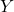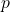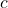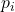# Complexity of Inference in Bayesian Networks

Developing efficient (i.e. polynomial time) algorithms with guaranteed performance is a central goal in computer science (perhaps the central goal). In machine learning, inference algorithms meeting these requirements are much rarer than we would like: often, an algorithm is either efficient but doesn’t perform optimally or vice versa. A number of results from the 1990’s demonstrate the challenges of, but also the potential for, efficient Bayesian inference. These results were carried out in the context of Bayesian networks.

Briefly, recall that a Bayesian network consists of a directed acyclic graph with a random variableat each vertex. Letbe the parents of. Then the Bayes net defines a distribution overof the form

(1)Inference in a Bayes net corresponds to calculating the conditional probability, whereare sets of latent and observed variables, respectively. Cooper  showed that exact inference in Bayes nets is NP-hard. (Here and in other results mentioned, the size of the problem is given by the total size of the probability tables needed to represent the Bayes net.) The reason the Cooper result holds is essentially that Bayes nets can be used to encode boolean satisfiability (SAT) problems, so solving the generic Bayes net inference problem lets you solve any SAT problem. But SAT is NP-complete, so Bayes net inference must be NP-hard.

A number of stronger and even more interesting results followed. First, Dagum and Luby  showed that even approximate inference is NP-hard. Specifically, let, defineandas before, and define. Then an absolute approximationsatisfies

(2)whileis a relative approximation if

(3)Dagum and Luby showed that finding either an absolute or relative approximation fordeterministically is NP-hard. Furthermore, finding either type of approximation with high probability using a randomized, polynomial time algorithm is impossible unless NPRP (RP is a randomized version of P, where on negative inputs the algorithm must always be correct but can be correct on positive inputs with only probability). There is good reason to believe this is not true because complexity theorists believe P = RP and PNP. Hence, we should not expect to be able to approximate conditional probabilities in arbitrary Bayes nets.

Roth  strengthened portions Dagum and Luby’s result by showing that relative approximation of Bayes nets is #P-complete. Recall that #P is the counting version of NP (instead of, “Is there a solution?”, the question is, “How many solutions are there?”). #P is believed to be a much harder class than NP. Indeed, a polynomial time algorithm with access to an oracle for a #P-complete problem can efficiently solve any problem in the polynomial hierarchy, which include NP, co-NP, and much more.

While these hardness of approximation results may appear disheartening, Dagum and Luby  were able to give a randomized, polynomial time algorithm which gives a relative approximation forunder one important, but very natural assumption. The proviso is that the conditional probabilities in the Bayes net are not extreme. Specifically, let

(4)and

(5)Define the local variance bound (LVB) to be the maximum value ofover all possible. The algorithm requires that the LVB be bounded by a polynomial of the size of the network. A nice property of the LVB requirement is that if eachfor some constantand any set of‘s, the LVB is still a constant, even if some of theare very small! The intuition for the LVB condition is that if you do have extreme probabilities, then you can have values “close” to 0 and 1, which would allow you to embed a SAT problem in the Bayes net. But if the ratio between the minimum and maximum conditional probabilities isn’t too large, then this won’t be possible.

There are a number of takeaways and issues these results raise, particularly if we think of Bayes nets as a prototype for Bayesian generative models in general. The positive results by Dagum & Luby based on excluding extreme probabilities suggests that one way to ensure tractability is to place restrictions on the distributions one considers instead of trying to work at the level structural constraints (though these may be necessary as well).  Furthermore, their results point to determinism being the enemy! As long as theare really random, inference in tractable. It’s only when theare close to being deterministic the problems arise. Although I didn’t mention it above,  both D&L and Roth’s results point toward the fact that it matters what questions you ask (i.e. which conditional probabilities you are interested in) –– some may be tractable to calculate while others may not be. And finally, perhaps we are limiting ourselves by asking for probabilities! Instead of calculating conditional probabilities, we could sample from the posterior (via, say, MCMC). Indeed, this is what D&L’s algorithm does, suggesting that sampling and calculating probabilities may be roughly equivalent in Bayes net world. However, this is not true in general. Indeed, under reasonable complexity assumptions, there are distributions that can be sampled in polynomial time but whose distribution cannot be computed efficiently . Thus, there are potentially interesting models with posteriors which can be sampled efficiently but not calculated efficiently.

References:

 Cooper, G. F., 1990. The computational complexity of probabilistic inference using Bayesian belief networks. Artif. Intell. 42, 393-405.
 Dagum, P. & Luby, M., 1993. Approximating probabilistic inference in Bayesian belief networks is NP-hard. Artif. Intell. 60, 141–153.
 Dagum, P. & Luby, M., 1997. An optimal approximation algorithm for Bayesian inference. Artif. Intell. 93, 1-27.
 Roth, D., 1996. On the Hardness of Approximate Reasoning. Artif. Intell. 82, 273-302.
 Yamakami, T., 1999. Polynomial time samplable distributions. J. of Complexity 15(4), 557-574.